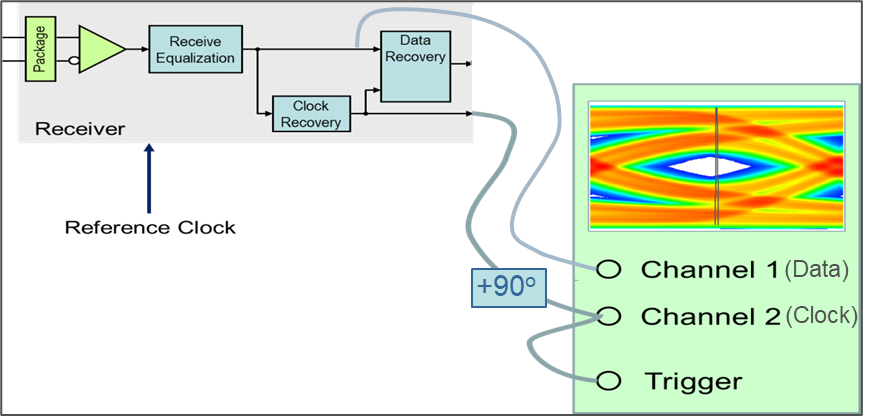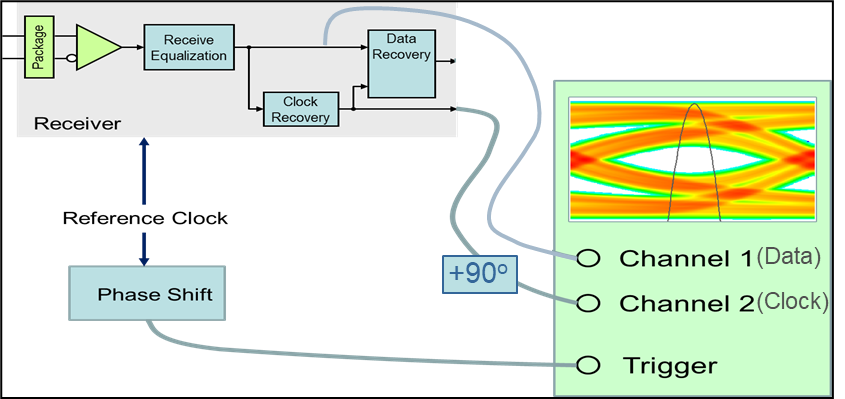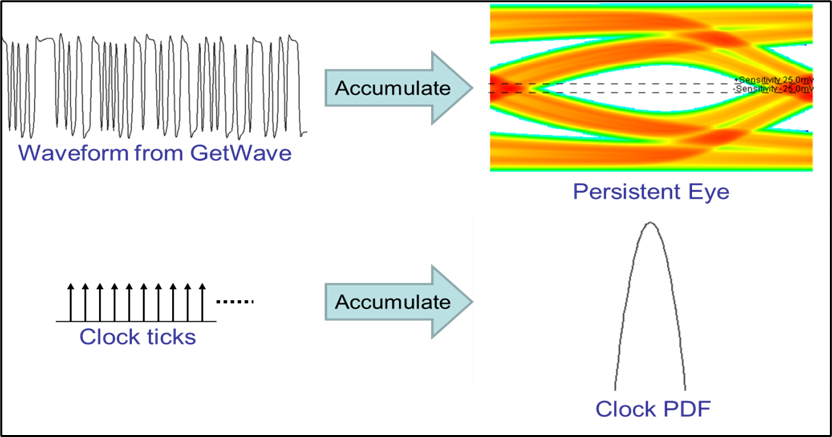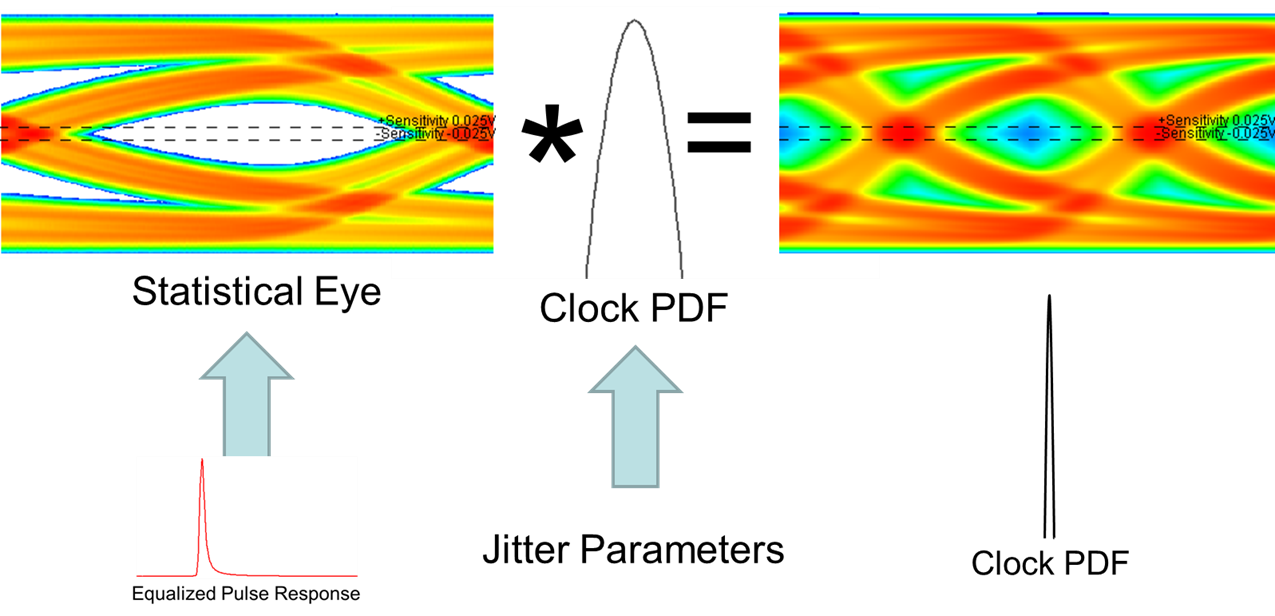## Clock Modes

The IBIS-AMI specification standardizes the way AMI models return waveform and clock data to an AMI simulator. But the standard says nothing about how the simulator should process that data. You can select from three different clock modes. Each clock mode is based on how the recovered clock and data distributions are used in the simulation to produce eye diagrams and predict bit error rates. Understanding and applying each of these modes effectively provides additional insight as to how, and where, a design’s operating margins are being affected and what to do about it. Each of the three clock modes are defined and presented in both time domain and statistical analysis along with their benefits and applicability.

Note

In Parallel Link Designer app, clock modes are only selectable when simulating in STAT mode. As such, the STAT mode box must be checked on all sheets that are to be simulated using a particular clock mode.

A serial link receiver design is the starting point to the understanding of QCD clock modes. The focus is on the relationship of the clock and data paths leading to the data decision latch.In a typical serial link receiver, the CDR is used to recover the clock signal from the serialized data stream. The recovered clock from the CDR does two things: it provides the clock signal that the sampling latch uses to capture the data, and it tells the DFE when to perform corrections to the data.

An ideal CDR having infinite bandwidth would provide a clock tick to the latch that is based on the data bit being latched. The dependency between the data and clock signals in this ideal CDR would be total. However, real world CDRs have limited bandwidth. Thus, the recovered clock being fed to the latch from the CDR is based upon a number of previous data bits. That means the clock tracks variations in the input signal, but only if they occur slowly enough for the CDR to respond to them. If the variations in the input edges are too fast for the CDR to respond, the CDR will track the only the average input rate and not respond to high frequency jitter. In this case, the variations in data and CDR behavior will be independent, as they both may vary but they won’t vary in unison. So, data and clock under the right circumstances can be treated as independent variables.

### Using Data and Clock Distribution to Predict Bit-Error Rate

The standard metric used to describe channel performance is the bit-error ratio (also known as BER). The clock and data distributions are what is used to determine the channel bit-error rate.The data bathtub curve (marked in black) is the data distribution at the data input to the Rx latch. The recovered clock (marked in red) is probed at the clock input to the Rx latch and shows a different distributed behavior. The sampling clock would ideally be in the center of the eye and its distribution is the result of multiple jitter sources. The bathtub curve is compared with the Rx clock PDF and the overlap between the curves determines the bit-error rate.

The BER equation is based on the product of the probability of error with a particular clock position and the probability of that clock position occurring. The values for all possible clock positions are then summed and the BER curve is plotted as shown. The highest probability calculated is the reported BER of the channel.

This view of the clock PDF and data bathtub can only be seen when looking at the sampling clock and data from the perspective of an ideal reference clock, such that the behaviors of clock and data are displayed independently. If clock and data are considered dependent, QCD will display the clock PDF as a delta function in the center of the UI because variation of the clock will be reflected in the eye diagram (data eye).

### Clock Modes

The three clock modes are: clocked, normal and convolved. These modes differ depending on the nature of the Rx clock recovery and assumptions of dependence, or independence between the clock and data.Clocked mode shows the data as it would be captured by the recovered clock. Normal mode al-lows the user to view both the data and clock independently with respect to an ideal external reference clock. Convolved mode combines the normal mode eye and clock PDF through con-volution to produce an eye diagram, and clock PDF that look as though the simulation were run in clocked mode. These clock modes are primarily designed for use in time domain simulations where the IBIS AMI model returns both the data waveform and clock ticks. Each of the modes will be discussed with regards to both time-domain and statistical analysis highlighting their benefits and when they are applicable for use.

### Time Domain Clock Mode: Clocked

The system representation of the clocked mode operation represents how the data is captured by the recovered clock from a system perspective.The data is captured at the input to the decision latch using the clock from the output of the clock recovery circuit. Note that the time values of AMI clocks are actually ½ UI before the data is sampled (at the start of the data bit), which makes them perfect to trigger waveform acquisition in the scope diagram. A ½ UI delay is added to the input of channel 2 to show the clock in proper relation to the input waveform.

The simulator operation in the clocked mode is shown:The simulated data waveform and clock is on the left and the resulting eye diagram and clock PDF that would be displayed as an output from the simulator is on the right. The clock PDF is displayed as a delta function in the center of the UI and the recovered clock variation due to noise and jitter is thus reflected in the data eye diagram. Because of this when in clocked mode it is not possible to distinguish where eye closure is coming from, whether it is in the data path or in the clock path. In this mode, clock and data are being treated as dependent variables, meaning the acquisition of waveform data is entirely dependent on the clock signals coming from the AMI model.

The clocked mode approach is intuitive from a systems perspective, because it emulates the way actual systems work. This is the way many AMI models have been designed to be used, and it is the only mode most other simulators support.

As one might expect, the calculation of BER in clocked mode is limited to the number of bits simulated. If there are only 1e6 samples of a process, one can only talk about probabilities down to 1e-6. This is the limitation of statistical significance that a user will incur in time domain simulations. To illustrate this the following example can be used:

If one could expect a time domain simulation to run at approximately one million bits per minute, after a minute a BER of 1e-6 could be produced. From a statistical significance standpoint this would be an insufficient sample size for most interface standards and proprietary designs. In general, a minimum sample size of 1e12, or a trillion bits, would be required to satisfy most current specifications. To simulate this many bits at a rate of one million bits per minute, the simulation would take almost 2 years. Under the right circumstances this limitation can be overcome by using normal clocked mode.

### Time Domain Clock Mode: Normal

Normal mode provides a method to extend the BER probabilities of time domain simulations into the ranges of most industry standards for channel design, and it does so without needing to use extrapolation algorithms. This is because normal mode is based on an assumption of independence between the recovered clock and the data at the Rx latch. While clocked mode treats clock and data as dependent processes, normal mode treats these distributions as independent. Because of this, the two independent distributions can be used to predict a significantly lower BER by estimating the probability of different interactions.

In normal mode, the data and clock are captured using an ideal reference clock source.This method of capture keeps the clock jitter and noise from affecting the data bathtub, and thus the data eye consists of the persistent waveform along with any channel ISI, and Tx jitter. The clock PDF starts with the accumulated probabilities of clock ticks returned by the model, which is then convolved with any jitter budgets from the model to create the presented clock PDF.

The simulator operation in the normal clocked mode is shown:The simulated data waveform and clock ticks is on the left and the resulting eye diagram and clock PDF that would be displayed is on the right. Unlike clocked mode, the data and clock distributions are accumulated independently. This provides a level of insight into model behavior that clocked mode cannot, namely how much of the eye closure is coming from the data path, and how much of the closure is due to jitter in the recovered clock signal. Also, jitter and noise sources are presented on the data bathtub and clock PDF separately, unlike clocked mode where clock jitter and noise distribution are only presented in the data bathtub.

Normal mode provides a method to predict lower bit-error-ratios by improving statistical significance of a time-domain simulation. In comparison with clocked mode, the assumption of independent clock and data allow the statistical distributions to be combined to predict overall probability. Referring back to the example of a time domain simulation taking one minute to predict a BER of 1e-6 in clocked mode, the combined distributions of a million data bits and a million clock bits would allow a BER prediction of 1e-12 in the same simulation time.

On the surface, this assumption of independence between clock and data may seem crazy. How could the recovered clock and data possibly be independent, when both come from the same source? It’s clear that they can’t be completely independent, otherwise the CDR would have no purpose. When independence is discussed in this context, what is really meant is jitter.

Specifically, this is the kind of low frequency, repetitive (usually sinusoidal) jitter that a CDR can track out. If the frequency of the jitter in the incoming signal is high or random enough, the CDR won’t follow it and the variations in data jitter and the recovered clock will be effectively statistically independent. Even when track-able jitter is present in the input signal, if its magnitude is low enough (say 1% of the UI or less) it’s impact on eye closure will be small enough that the additional statistical significance of normal mode may be worth the price. When there is significant track-able sinusoidal jitter, the CDR’s ability to remove track-able jitter is not accounted for, and the effect of that jitter is effectively double counted, making the simulation results pessimistic. How pessimistic depends on the magnitude and frequency of the incoming sinusoidal jitter. Under these conditions normal mode would not be a useful predictor of BER.

### Time Domain Clock Mode: Convolved

The third clock mode is convolved. The primary function of convolved mode is to validate assumptions of data and clock independence. This is basically a validation of the normal mode simulation.In time domain with the clock mode set to convolved, the data eye and clock PDF captured in normal mode are convolved together to present an eye and clock that look as though the simulation had been run in clocked mode. If the jitter processes in the data path and the clock recovery path are reasonably independent, the eye diagram from a convolved mode simulation will look the same as the eye from a clocked mode simulation. This means that the clock recovery process essentially involves no tracking of the data, the clock and data are independent, and normal mode can be used to reliably predict BERs lower than are possible by clocked mode.

### Statistical Simulations and Clock Modes

Statistical simulations are based solely on the impulse response of the channel and data pattern dependencies. There is no waveform nor returned clock ticks from the model in statistical simulations. Because no clock is returned from the model clocked mode and convolved mode are identical.

The clock position in a statistical analysis simulation is determined by an algorithm called “Hula Hoop”. This algorithm places a ring 1UI wide over the equalized pulse response of the channel. The data eye is represented by the time points where the ring touches the pulse response. The center point is where the clock PDF is placed.

Statistical analysis in normal mode produces an eye diagram that is the product of the equalized pulse response and probabilities of bit switching.The ISI of the channel is determined directly from the equalized pulse response and through mathematical analysis the eye diagram can be obtained which includes any Tx jitter. Rx Jitter and Rx clock recovery jitter are IBIS-AMI parameters that are specified or embedded in the AMI model and will be imposed on the clock PDF.

Clocked and convolved modes are performed the same way in statistical analysis. The statistical eye generated using the equalized pulse response is convolved with the clock PDF which is based on the Rx jitter and Rx clock recovery jitter parameters.### Setting Clock Mode

The clock mode can be set from the Serial Link Designer or Parallel Link Designer by accessing the Designator Element properties dialog box. To open the dialog box, double click on either a Tx or Rx designator on the schematic sheet, The clock mode column in the dialog window is a pull-down menu allowing you to select a clock mode for the Rx. You can opt to use the check box next to the pull-down selector to sweep clock modes. If this box is checked, the clock mode becomes a variable in the solution space for the schematic sheet. You can then set and sweep the various modes.